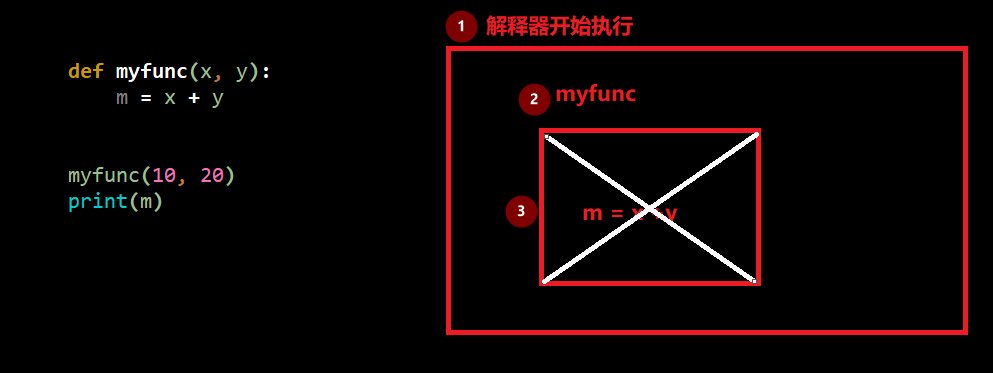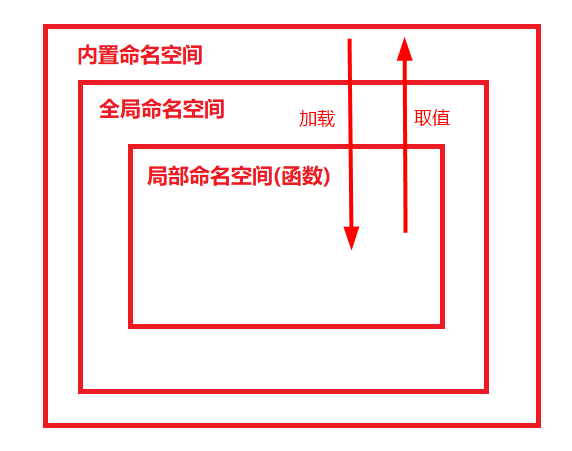# 🐴Python之路—函数进阶✔️

1. 懒惰

1. 缺乏耐性

1. 傲慢

## 🍗 药引

``````def myfunc(x, y):
m = x + y

myfunc(10, 20)
print(m)
``````

so​ 😕, 执行代码：

``````NameError: name 'm' is not defined
````````````1. 从Python解释器开始执行之后，就会在内存中开辟一个空间
2. 每当遇到一个变量时，就会把变量名和值的对应关系保存下来
但是当遇到函数定义时，解释器只是象征性的把函数名读入内存，表示知道有这个函数存在了，而函数内部的变量和逻辑，解释器不关心。
3. 等调用函数时，python解释器会在开辟一块内存来存储函数里的内容，这个时候才关注函数里面有哪些变量，而函数中的变量只能在函数内部使用，并且会随着函数执行完毕，这块内存中的内容会被清空（垃圾回收）
``````

## 命名空间

• 全局命名空间—代码在运行伊始，创建的存储“变量名与值的关系”的空间
• 局部命名空间—在函数的运行中开辟的临时的空间
• 内置命名空间—程序运行前加载

PS:*内置命名空间中存放了python解释器为我们提供的名字：input,print,str,list,tuple…它们都是我们拿过来就可以用的方法。

## 作用域

• 全局作用域：包含内置名称空间、全局名称空间，在整个文件的任意位置都能被引用、全局有效
• 局部作用域：局部名称空间，只能在局部范围内生效

### global

global关键字的作用：全局声明

``````a = 10
def func():
global a
a = 20

print(a)
func()
print(a)
``````

## 函数嵌套

### 函数嵌套定义

``````def f1():
print("in f1")
def f2():
print("in f2")

f2()
f1()
``````

### 函数嵌套调用

``````def max2(x, y):
'''比较两个数的大小'''
m = x if x > y else y
return m

def max4(a, b, c, d):
res1 = max2(a, b)
res2 = max2(res1, c)
res3 = max2(res2, d)
return res3

the_max = max4(23, -7, 30, 11)
print(the_max)

``````

## 函数名

``````def func():
print('in func')

print(func)		# output:<function func at 0x0000019B7FF22EA0>
``````
1. 可以被引用

``````def func():
print('in func')

f = func
print(f)
``````
2. 可以被当作容器类型的元素

PS: 一句话，可以当成普通变量用

## 闭包

``````def func():  # 外部函数
name = 'mark'
def inner():  # 内部函数
print(name)
print(inner.__closure__)   #判断闭包函数的方法
return inner

f = func()
f()

``````

## 总结嵌套定义：定义在内部的函数无法直接在全局被调用

就是一个变量，保存了函数所在的内存地址

内部函数包含对外部作用域而非全剧作用域名字的引用，该内部函数称为闭包函数